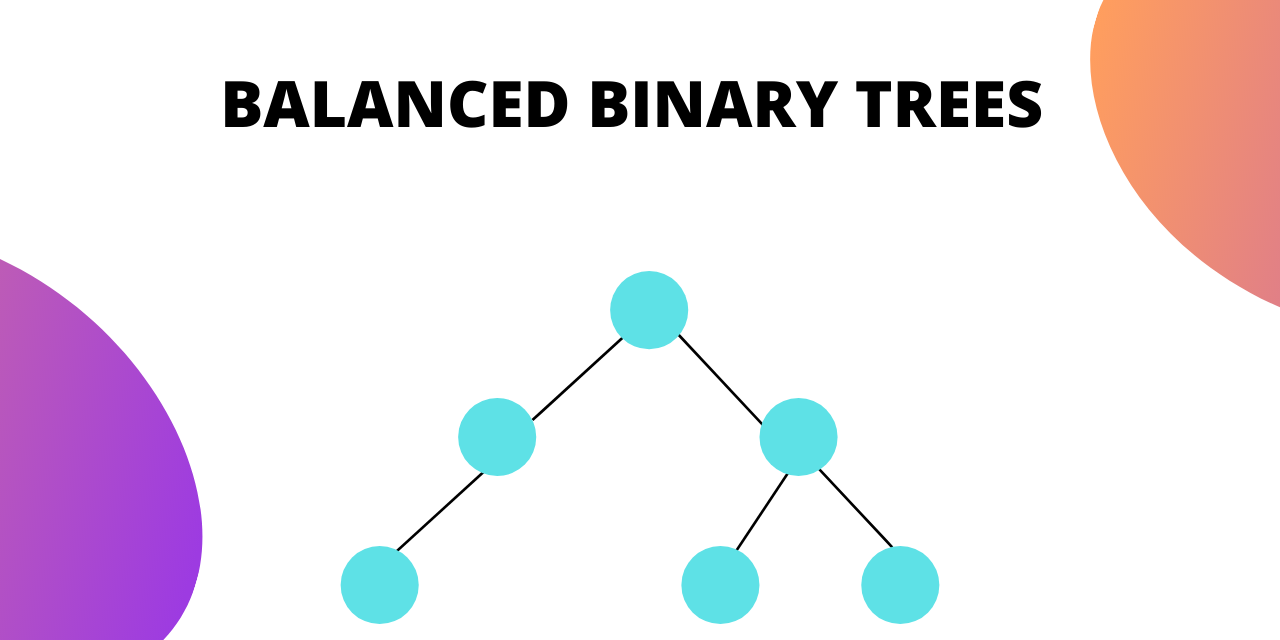# What is a Balanced Binary Tree and How to Check it?

Published on August 3, 2022By Jayant VermaWhile we believe that this content benefits our community, we have not yet thoroughly reviewed it. If you have any suggestions for improvements, please let us know by clicking the “report an issue“ button at the bottom of the tutorial.

In case of binary trees, if the trees are skewed, they become computationally inefficient to perform operations on.

This is the motivation behind making sure that trees are not skewed. Hence the need for balanced binary trees.

## What is a Balanced Binary Tree

Balanced Binary trees are computationally efficient to perform operations on.

A balanced binary tree will follow the following conditions:

1. The absolute difference of heights of left and right subtrees at any node is less than 1.
2. For each node, its left subtree is a balanced binary tree.
3. For each node, its right subtree is a balanced binary tree.

## Height-balanced Binary Trees

Balanced binary trees are also known as height-balanced binary trees. Height balanced binary trees can be denoted by HB(k), where k is the difference between heights of left and right subtrees. ‘k’ is known as the balance factor.

If for a tree, the balance factor (k) is equal to zero, then that tree is known as a fully balanced binary tree. It can be denoted as HB(0).

## Self Balancing Binary Search Tree

If a binary search tree has a balance factor of one then it is an AVL ( Adelso-Velskii and Landis) tree. This means that in an AVL tree the difference between left subtree and right subtree height is at most one.

AVL tree is a self-balancing binary search tree. In an AVL tree if the difference between left and right subtrees is greater than 1 then it performs one of the following 4 rotations to rebalance itself :

• Left rotation
• Right rotation
• Left-Right rotation
• Right-Left rotation

## How to Check if a Binary Tree is balanced?

To check if a Binary tree is balanced we need to check three conditions :

1. The absolute difference between heights of left and right subtrees at any node should be less than 1.
2. For each node, its left subtree should be a balanced binary tree.
3. For each node, its right subtree should be a balanced binary tree.

We will need a function that can calculate the height of the tree. One way to do this is to write a separate function for calculating the height and call it every time height is needed. This is going to be computationally inefficient.

The better way to implement this will be returning height in the same function.

For each node, we will return -1 if it is not balanced and the height of that node/subtree if it is balanced.

The algorithm is as follows :

• If node == null -> return 0
• Check left subtree. If not balanced -> return -1
• Check right subtree. If not balanced -> return -1
• The absolute between heights of left and right subtrees. If it is greater than 1 -> return -1.
• If the tree is balanced -> return height

The pseudo code will look like as follows :

`````` private int balanceHeight (TreeNode currentNode)
{
if (currentNode == null)
{
return 0;
}

// checking left subtree
int leftSubtreeHeight = balanceHeight (currentNode.left);
if (leftSubtreeHeight == -1) return -1;
// if left subtree is not balanced then the entire tree is also not balanced

//checking right subtree
int rightSubtreeHeight = balanceHeight (currentNode.right);
if (rightSubtreeHeight == -1) return -1;
// if right subtree is not balanced then the entire          tree is also not balanced

//checking the difference of left and right subtree for current node
if (Math.abs(leftSubtreeHeight - rightSubtreeHeight) > 1)
{
return -1;
}
//if it is balanced then return the height
return (Math.max(leftSubtreeHeight, rightSubtreeHeight) + 1);
}
``````

You can call this function on the root of the tree and if it returns anything other than -1 then it is a balanced binary tree. If it returns -1 then it is not a balanced binary tree.

## Conclusion

In this tutorial we covered Balanced binary trees and how can we check if a tree is balanced binary tree. Hope you understood and enjoyed learning with us.

Thanks for learning with the DigitalOcean Community. Check out our offerings for compute, storage, networking, and managed databases.Jayant Verma

author

#### Still looking for an answer?

Ask a questionSearch for more help

Click below to sign up and get \$200 of credit to try our products over 60 days!

### Popular Topics

##### Join the Tech Talk##### Hollie's Hub for Good

Working on improving health and education, reducing inequality, and spurring economic growth? We’d like to help.